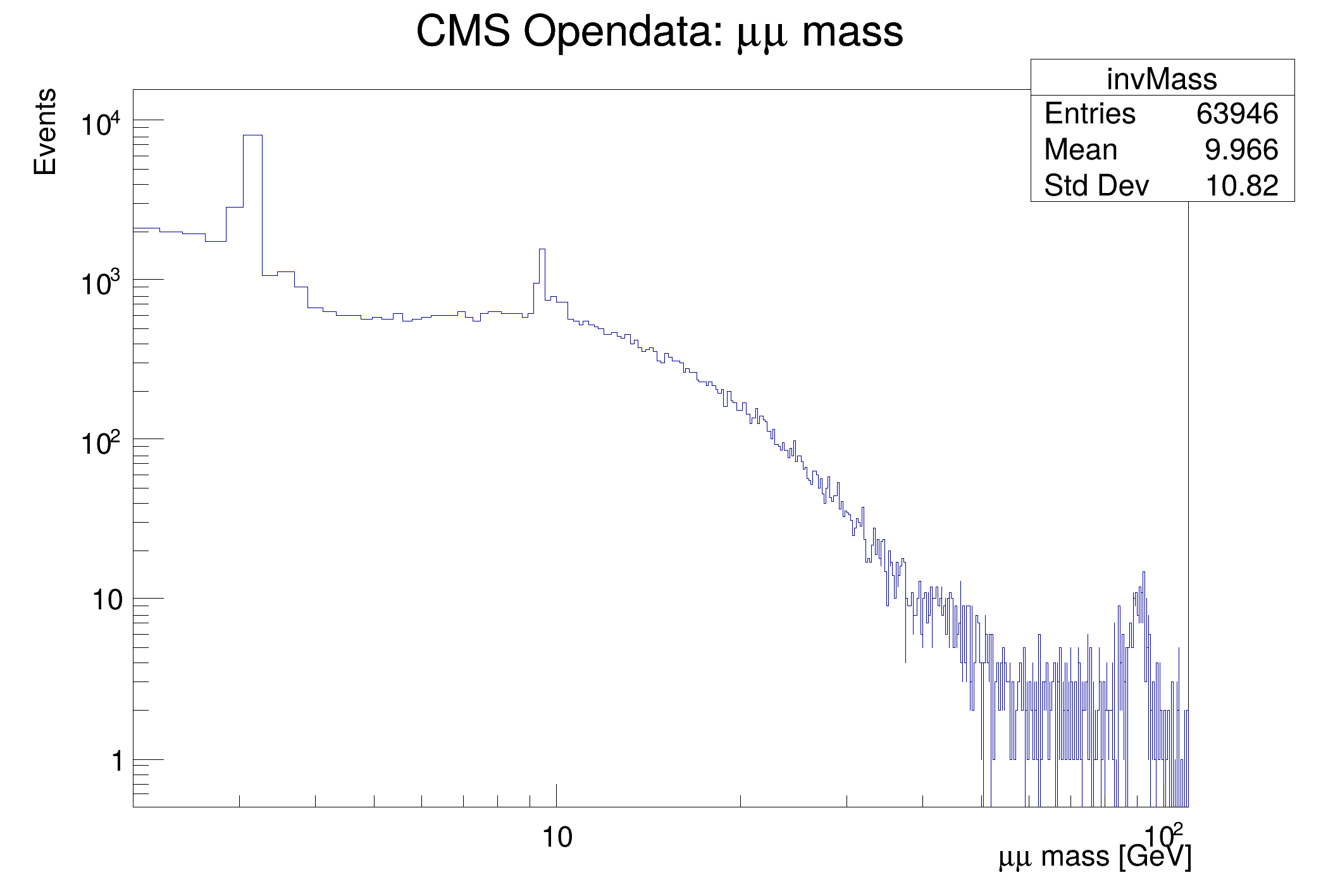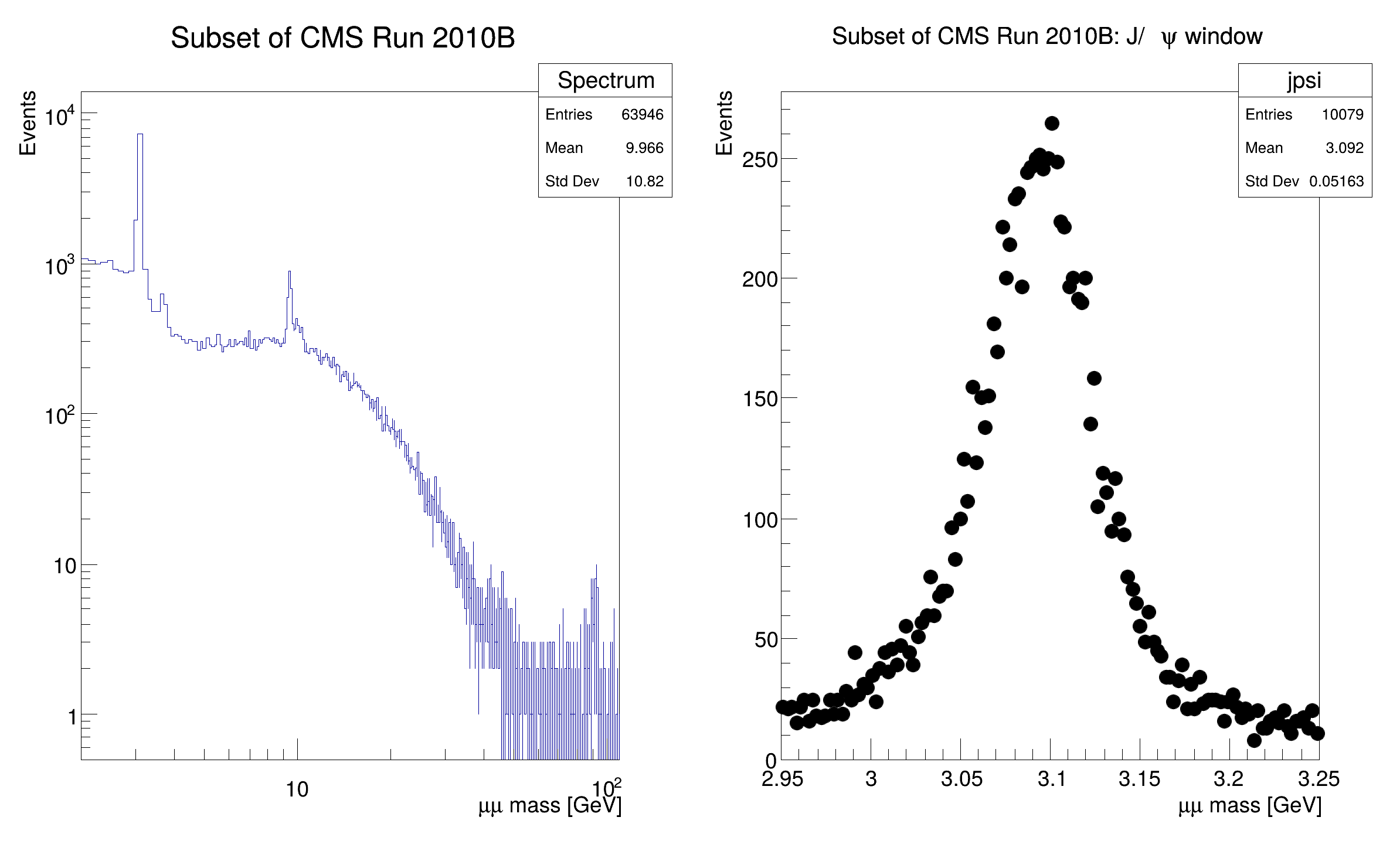ROOT   Reference Guidedf014_CSVDataSource.C File Reference

## Detailed Description

Process a CSV file with RDataFrame and the CSV data source.This tutorial illustrates how use the RDataFrame in combination with a RDataSource. In this case we use a RCsvDS. This data source allows to read a CSV file from a RDataFrame. As a result of running this tutorial, we will produce plots of the dimuon spectrum starting from a subset of the CMS collision events of Run2010B. Dataset Reference: McCauley, T. (2014). Dimuon event information derived from the Run2010B public Mu dataset. CERN Open Data Portal. DOI: 10.7483/OPENDATA.CMS.CB8H.MFFA.

{
// Let's first create a RDF that will read from the CSV file.
// The types of the columns will be automatically inferred.
auto fileNameUrl = "http://root.cern.ch/files/tutorials/df014_CsvDataSource_MuRun2010B.csv";
auto fileName = "df014_CsvDataSource_MuRun2010B_cpp.csv";
if(gSystem->AccessPathName(fileName))
TFile::Cp(fileNameUrl, fileName);
auto df = ROOT::RDF::MakeCsvDataFrame(fileName);
// Now we will apply a first filter based on two columns of the CSV,
// and we will define a new column that will contain the invariant mass.
// Note how the new invariant mass column is defined from several other
// columns that already existed in the CSV file.
auto filteredEvents =
df.Filter("Q1 * Q2 == -1")
.Define("m", "sqrt(pow(E1 + E2, 2) - (pow(px1 + px2, 2) + pow(py1 + py2, 2) + pow(pz1 + pz2, 2)))");
// Next we create a histogram to hold the invariant mass values and we draw it.
auto invMass =
filteredEvents.Histo1D({"invMass", "CMS Opendata: #mu#mu mass;#mu#mu mass [GeV];Events", 512, 2, 110}, "m");
auto c = new TCanvas();
c->SetLogx();
c->SetLogy();
invMass->DrawClone();
// We will now produce a plot also for the J/Psi particle. We will plot
// on the same canvas the full spectrum and the zoom in the J/psi particle.
// First we will create the full spectrum histogram from the invariant mass
// column, using a different histogram model than before.
auto fullSpectrum =
filteredEvents.Histo1D({"Spectrum", "Subset of CMS Run 2010B;#mu#mu mass [GeV];Events", 1024, 2, 110}, "m");
// Next we will create the histogram for the J/psi particle, applying first
// the corresponding cut.
double jpsiLow = 2.95;
double jpsiHigh = 3.25;
auto jpsiCut = [jpsiLow, jpsiHigh](double m) { return m < jpsiHigh && m > jpsiLow; };
auto jpsi =
filteredEvents.Filter(jpsiCut, {"m"})
.Histo1D({"jpsi", "Subset of CMS Run 2010B: J/#psi window;#mu#mu mass [GeV];Events", 128, jpsiLow, jpsiHigh},
"m");
// Finally we draw the two histograms side by side.
auto dualCanvas = new TCanvas("DualCanvas", "DualCanvas", 800, 512);
dualCanvas->Divide(2, 1);
fullSpectrum->DrawClone("Hist");
dualCanvas->cd(2);
jpsi->DrawClone("HistP");
return 0;
}Date
October 2017

Definition in file df014_CSVDataSource.C.

c
#define c(i)
Definition: RSha256.hxx:119
m
auto * m
Definition: textangle.C:8
df014_CSVDataSource
Definition: df014_CSVDataSource.py:1
TFile::Cp
virtual Bool_t Cp(const char *dst, Bool_t progressbar=kTRUE, UInt_t buffersize=1000000)
Allows to copy this file to the dst URL.
Definition: TFile.cxx:4843
TSystem::AccessPathName
virtual Bool_t AccessPathName(const char *path, EAccessMode mode=kFileExists)
Returns FALSE if one can access a file using the specified access mode.
Definition: TSystem.cxx:1294
ROOT::RDF::MakeCsvDataFrame